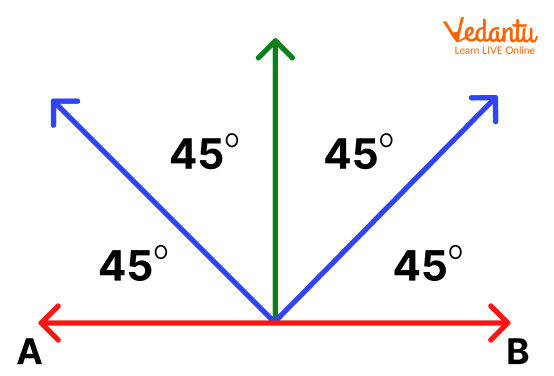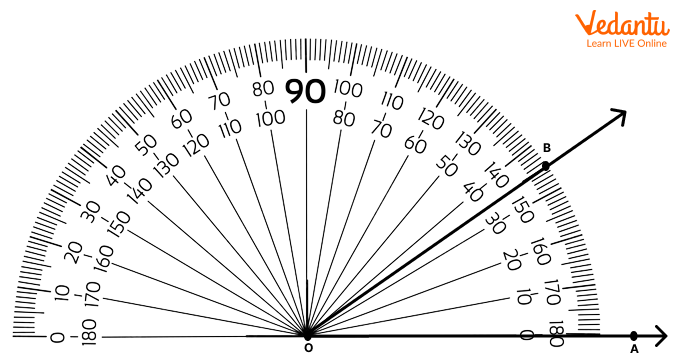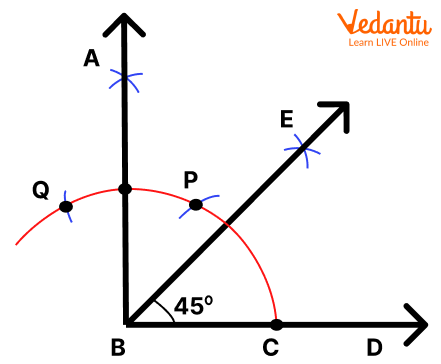Courses
Courses for Kids
Free study material
Free LIVE classes
More

# 45 Degree AngleLIVE
Join Vedantu’s FREE Mastercalss

## What is a 45 Degree Angle?

Before starting with the definition of a 45 degree angle, let us first understand the meaning of an angle. When two rays are joined at a common point, they form an angle. The common point is known as the node or vertex and the two rays form the arms of an angle.  ‘∠’ is the symbol that is used to represent an angle and it is generally measured in degree (°). 30°, 45°, 60°, 90°, and 180° are some commonly known degree angles.

A 45-degree angle can be defined as an acute angle that is formed by bisecting a 90 degree angle into two equal halves. Each half of the 90 degree angle is equal to 45 degrees. To help you understand better, we have also provided a few examples of 45 degree angles and also the step-by-step construction of 45 degree angles.

Look at the 45 degree angle picture that is given below for better understanding.## How can We Construct a 45 Degree Angle?

Constructing a 45 degree angle is very easy. It can be constructed with the help of a protractor or a compass. Here, we have provided a step-by-step method to construct a 45-degree angle using both a protractor and a compass.

## Constructing a 45-Degree Angle Using a Protractor

You can draw a 45 degree angle easily by following the steps given below. We have also provided a picture below to help you understand better.

• Let us first start by drawing a line segment OA.

• Next, place the protractor at point O in such a way that the reading 90° is perpendicular to point O.

• Now, you will have to find the reading for 45°. Once you find it, mark a dot at the 45° reading with a pencil and name it B.

• Remove the protractor and draw a line using a scale to join the points O and B.

• An angle is formed (∠AOB), which is equal to 45°.## Constructing a 45 Degree Angle with a Compass

Drawing a 45 degree angle with a compass might look a little more difficult than the protractor method. But if you follow the correct steps, then it is fun and easy.

Refer to the below-given picture carefully and then follow the instructions given below:1. Let us first draw a straight line, BD.

2. Now, place the tip of the compass at point B. Take a width which should be more than half of BD.

3. We need to draw an arc which intersects the line BD at a point. Mark this point as D.

4. Take the same width as mentioned above and place the tip of the compass at point D. Carefully, cut the arc drawn in step 3 and name it as point P.

5. Now, put the tip of the compass at point P and make another cut to the arc. Name this as point Q.

6. Keeping the compass at the same point, i.e., point P, draw another arc perpendicular to point B.

7. Repeat the same step from point Q. Mark the point of intersection of both the arcs as A.

8. Join both points A and B with a straight line. We now get a 90 degree angle, ∠ABD.

9. Since we need a 45 degree angle, we will have to bisect the 90 degree angle. Keeping the compass tip at point C, draw an arc anywhere between ∠ABD.

10. Now, place the compass tip at point A and cut the previous arc which you have drawn between ∠ABD. Name their intersection point as E. Join the points E and B.

Finally, we get our required 45 degree angle, i.e., ∠EBD. We hope you had fun while constructing a 45 degree angle. Although it has a lot of steps and many students can find it difficult, it will be really easy to draw if you practise it regularly.

## Examples of 45 Degree Angles in Real Life

Now, after going through this article, you got an idea about the 45 degree angle and how it looks. Let us now see some real-life examples of 45 degree angles. If we observe properly, we can find out that most of the things in our surrounding areas form a 45 degree angle. For example, a door can form a 45 degree angle when kept open or even our laptop lid if we hold it properly in a particular manner.

You can find a few more examples below:

• Many solar panels are constructed at an inclination of 45 degree angle as the sun's rays reach the farthest at this angle.

• It is also used by many architects while constructing doors, window grills, and buildings.

## Conclusion

We hope this article helped you in learning about 45 degree angles and how we can construct them easily. While constructing, we must also be very careful about the measurement and drawing of the arc and rays to avoid any misinterpretation of the result. You can also find out the procedure to draw different angles on our website. This is an important topic under Geometry and regular practice will help you to draw any angle easily in your examination.

Last updated date: 29th Sep 2023
Total views: 141.3k
Views today: 4.41k

## FAQs on 45 Degree Angle

1. Explain what does a 45 degree angle look like with examples.

One best example for a 45 degree angle is a scissor. The open face of a scissor perfectly resembles the angle of 45 degrees. Even we can take the example of greater than sign (>) or even lesser than sign (<) to show what does a 45 degree angle look like.

2. Is a 45 degree angle an acute angle?

Yes, 45 degree angle is an example of an acute angle. Any angle that measures less than 90 degrees is called an acute angle. If we divide a 90 degree angle through the angular point, we get two angles of 45 degrees each.